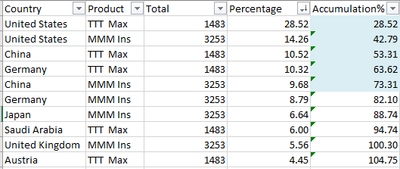# Qlik Sense App Development

Announcements
Coming your way, the Qlik Data Revolution Virtual Summit. October 27-29. REGISTER
cancel
Showing results for
Did you mean:
HighlightedCreator III

## Background color for accumulation of Percentage values

I need background color for each line in the table based on Percentage accumulation reaches till 50% then Green and rest are Yellow. How to achieve this?Labels (1)
• ### Qlik Sense

1 Solution

Accepted Solutions
HighlightedCreator III

Hi,

I found this solution and it works perfectly. Thanks for you effort 🙂

=if(rangesum(above(total sum(IB_COUNT)/sum(Total IB_COUNT),0,RowNo(Total)))<=0.81, rgb(179, 198, 255),
if( rangesum(above(total sum(IB_COUNT)/sum(Total IB_COUNT),0,RowNo(Total)))>=0.80, rgb(255, 255, 204)))

9 Replies
HighlightedCreator II

Hi,

Try this;

``````Aggr(
If(Rangesum(Above(Sum(Percentage),1,RowNo()))<=0.5, green(),yellow()),
(Country, [Target Product],(=Sum(Percentage),Desc))
)``````
HighlightedCreator III

Hi,

It's not working for me. I've attached the data for your reference. please let me know if you get any solutions.

HighlightedCreator II

Hi,

First, you should add row_number field with RecNo() at script level. Then you can use below expression to do the trick;

``````Aggr(
If(Rangesum(Above(Sum(Percentage),1,RowNo()))<=0.5, green(), yellow()),
(rn,(=Sum(Percentage),Desc))
)
``````

I will upload the sample .qvd when i go home.

HighlightedCreator III

Hi,

HighlightedCreator II

Hi,

Check the attached app.

HighlightedCreator III

Hi, Still not getting the expected result. I've the Percentage Expression as =sum(IB_COUNT)/sum(total IB_COUNT) and I used the below format as your said.

Aggr(

If(Rangesum(Above(sum(IB_COUNT)/sum(total IB_COUNT)),1,RowNo()))<=0.5, green(), yellow()

),(rn,(=(sum(IB_COUNT)/sum(total IB_COUNT)),Desc)))

Need help on this. Thanks!

HighlightedCreator II

Can you share the expected result as excel or image?

HighlightedCreator III

Hi,

Thanks for your response so far!

See the below image of which Blue color Background is should be <=80% . Percentage column is sort by largest to smallest.HighlightedCreator III

Hi,

I found this solution and it works perfectly. Thanks for you effort 🙂

=if(rangesum(above(total sum(IB_COUNT)/sum(Total IB_COUNT),0,RowNo(Total)))<=0.81, rgb(179, 198, 255),
if( rangesum(above(total sum(IB_COUNT)/sum(Total IB_COUNT),0,RowNo(Total)))>=0.80, rgb(255, 255, 204)))